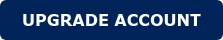Filter Design for Grid Connected Systems

Nowadays, environmental concerns are pushing society towards renewable and more efficient energy sources. The utilization of the aforementioned sources is made possible by using switching power conversion devices. However, in order to ensure a good quality of the grid voltage, it is essential to introduce a low-pass filter after the inverting stage.

The objective of this App Note is to show how to design a filter for grid-connected inverters that are widely used in solar and wind generation plants. Namely, various topologies and strategies to optimize the size, stability, and efficiency of the system will be covered.

Tolopoly Selection

L-Filter:

• First-order filter with attenuation 20 dB/decade
• Suitable for a converter with a high switching frequency
• Decreases dynamics of the whole system

LC-Filter:

• Second-order filter with attenuation 40 dB/decade
• The resonant frequency of the filter is dependent on the grid

LCL-Filter:

• Attenuation of 60 dB/decade for frequencies above the resonant frequency
• Better decoupling between the filter and the grid impedance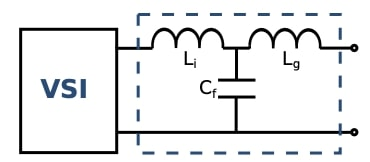Design Procedure for LCL Filters:

LCL topology is selected for grid-connected inverter filters due to its advantages described in the topology selection section.Filter capacitance should be designed for the maximum grid power factor variation of 5%. (x=0.05)

Inverter side inductor is designed to limit the ripple current to a certain amount, normally 10% of the rated current. (k=0.1)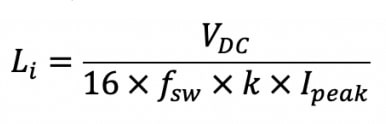Where Sn is the rated apparent power, and Vn is the line to line RMS voltage. The grid side inductor is designed to limit the dominant harmonic current amplitude to the specified limit.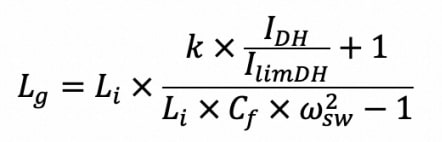Where:

• IDH is the amplitude of the dominant harmonic
• IlimDH is the desired amplitude at the dominant harmonic

FFT Analysis and Results: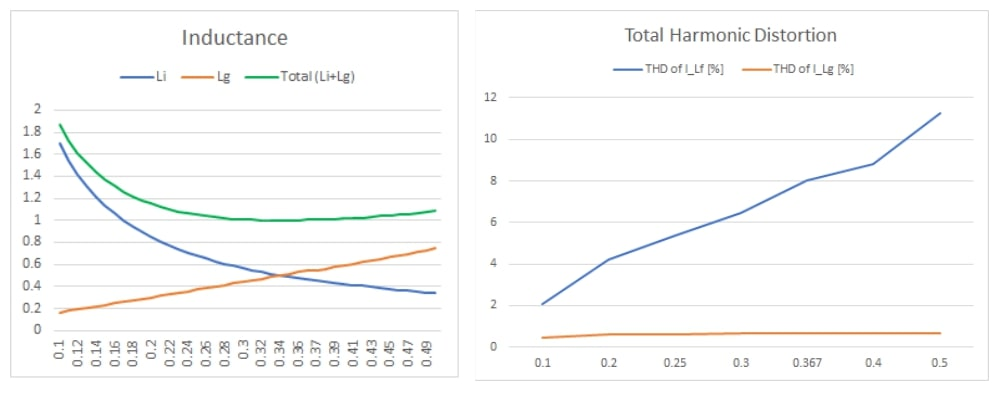These figures show how changing the value of k, it is possible to tailor the filter for specific needs. The figure on the left demonstrates how values of the grid side and inverter side inductances change with k from 0.1 to 0.5, with the minimum total inductance appearing at k=0.34. However, the figure on the right shows that total harmonic distortion on the inverter side increases with the increasing k, as expected. So, using this method, the design of an LCL filter can be optimized based on the desired size relation between inductors, and allowable THD and power losses.

Conclusions

In high power, grid-connected applications, the size, efficiency, and cost of a filter, as well as the total harmonic distortion, can be of foremost importance. After learning from the described design procedure and its implementation, Frenetic's artificial intelligence can obtain the most optimal filter design along with the advanced magnetic design of inductors for your application.

You may also like